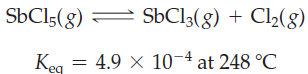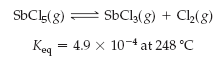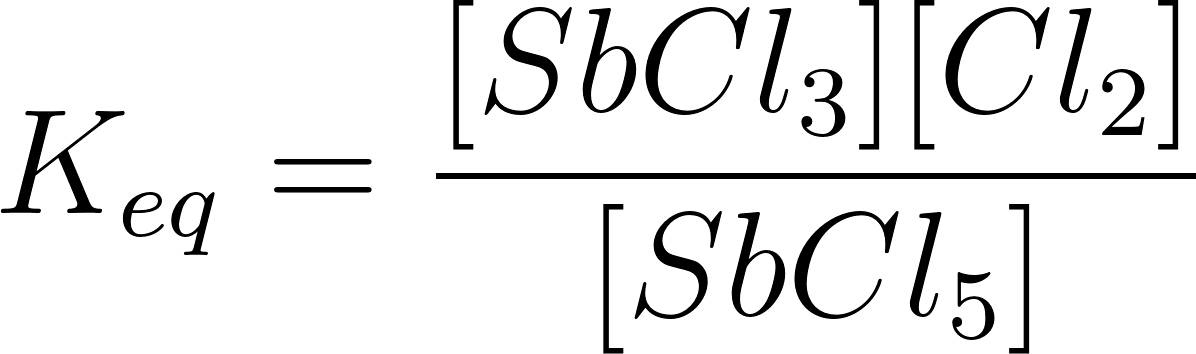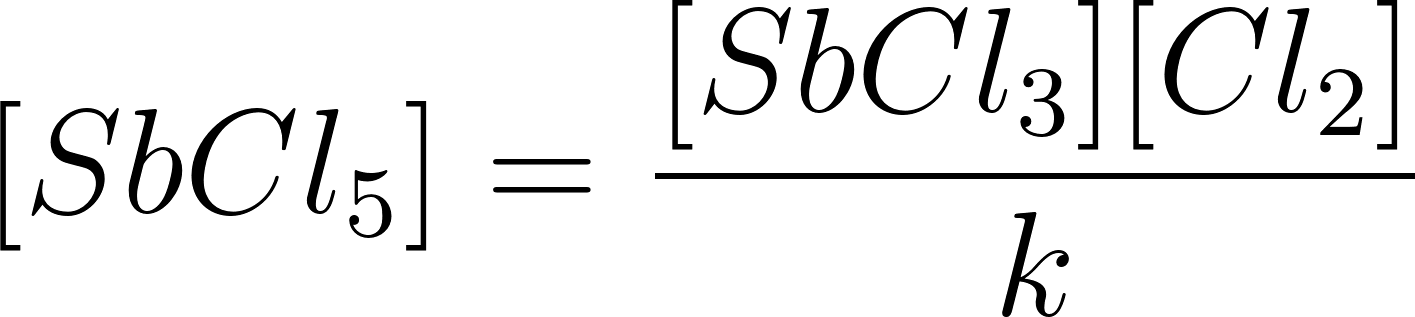×
Get Full Access to Introductory Chemistry - 5 Edition - Chapter 15 - Problem 57p
Get Full Access to Introductory Chemistry - 5 Edition - Chapter 15 - Problem 57p

×

# An equilibrium mixture of the following reaction is found to have [SbCl3] = 0.0255 M andISBN: 9780321910295 34

## Solution for problem 57P Chapter 15

Introductory Chemistry | 5th Edition

• Textbook Solutions
• 2901 Step-by-step solutions solved by professors and subject experts
• Get 24/7 help from StudySoup virtual teaching assistantsIntroductory Chemistry | 5th Edition

4 5 1 361 Reviews
26
5
Problem 57P

An equilibrium mixture of the following reaction is found to have [SbCl3] = 0.0255 M and [Cl2] = 0.135 M at 248 °C. What is the concentration of SbCl5?Step-by-Step Solution:

Problem 57P

An equilibrium mixture of the following reaction is found to have [SbCl3] = 0.0255 M and [Cl2] = 0.135 M at 248 °C. What is the concentration of SbCl5?Step by step solution

Step 1 of 2

The given equilibrium reaction is as follows.The equilibrium constant for the reaction is as follows.Rearrange the equilibrium expression......................(1)

Step 2 of 2

##### ISBN: 9780321910295

Unlock Textbook Solution